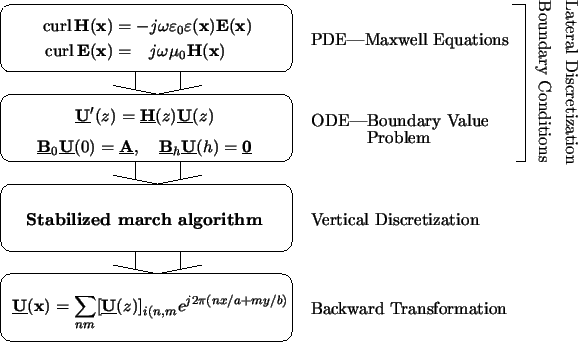Next: 6.5.1 Performance Up: 6. Differential Method Previous: 6.4.3.2 Multiple Shooting

# 6.5 Discussion

In this section we discuss the performance of the differential method. We will first estimate the numerical and storage demands, which are governed by the vertical solution of the BVP since all other operations imply basically two-dimensional Fourier transformations that can be evaluated with the FFT. Then some limitations of the differential method are described and compared to the second frequency domain method of the waveguide model.

Before we start this discussion we summarize the basic four steps of the differential method in Figure 6.5. The first two steps refer to the transformation of the Maxwell equations into a BVP and are mainly of theoretical interest. The numerical efficiency is exclusively determined by the third step, i.e., by the performance of the stabilized march algorithm, since the last step of the backward transformation is a simple two-dimensional Fourier transformation.Heinrich Kirchauer, Institute for Microelectronics, TU Vienna
1998-04-17